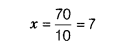# The perimeter of a triangle is 70 cm and its sides are in the ratio 2:3:5

The perimeter of a triangle is 70 cm and its sides are in the ratio 2:3:5.Find the length of sides of triangle.

Given, perimeter of a triangle = 70 cm
and ratio of its sides is 2 : 3 : 5
Let the sides of a triangle be 2x , 3x and 5x.
Perimeter of triangle=2x+3x+5x

70=10xSo, the sides are 2x=2x7=14,3x=3x7=21
and 5x=5x7=35